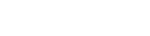# Operators

These are mostly used in calculations, comparisons, and operations on binary bits.

They are evaluated in order of importance (precedence).

For example, in a calculation: times (x) takes precedence over plus (+) so 2 + 3 x 2 equals 8 rather than 10. A good calculator should obey this precedence rule. For an exercise: check it on your smartphone calculator app.

The following tables list operators with the highest precedence first.

## Mathematical Operators

Operator Description
-x Negation
* / % Times / Divide / Remainder
- Subtract

The remainder is applied to integers and works like this: 5 % 2 equals 1 because 5 divided by 2 equals 2 remainder 1.

One good use for this operator (otherwise known as the modulo operator) is to limit the range of a counter value. If the divisor is n, then the counter will count between 0 and n - 1, rolling back to zero on each multiple of n. Look forward to a code example using this concept later in the tutorial series.

Some assignment shortcuts are available as follows (these have the lowest precedence over anything - as you might assume given that they are accepting the result of a prior evaluation):

Example Equivalent Operation
x += 1 x = x + 1
x -= 1 x = x - 1
x *= 2 x = x * 2
x /= 2 x = x / 2
x %= 3 x = x % 3

## Boolean Operators

These are the comparison, and logical operators that are mainly used in if statements.

Operators Description
< == > != >= <= Comparison operators
! not NOT
&& and AND
|| or OR

A common typo error is to enter just one equals sign meaning assign rather than compare, but the editor will detect this and signal a warning to you.

The result of a boolean operation is true or false.

Example: x = 3 > 4 or 5 < 6 # x == true

## Bitwise Operators

Note: bitwise operators are not likely to be used very often unless you are doing something quite technical involving bit manipulation.

Every number or character consists of binary bits, so we are able to flip bits, shift bits, mask bits etc. Individual bits are zeros and ones. They are set or reset according to logical operations related to how digital logic gates operate.

The first bit is called the LSB (Least Significant Bit) and the last bit is called the MSB (Most Significant Bit). Numbers constructed from zero or one bits are binary numbers.

Counting in binary goes like this: 0 1 10 11 100 101 110 111 1000 …

However, in the editor, we only see decimal equivalent numbers. So you will need to imagine the binary equivalent of 8 is 1000.

For an exercise: use the programmer’s calculator in Windows (or the equivalent app in your OS) to get used to fooling around with binary numbers.

Operator Description
~ NOT (inverts the bits)
<< >> Shift bits of left-side operand left or right by n positions (1 << 2 == 4)
& Logical AND of two values
^ Logical XOR of two values
| Logical OR of two values
&= |= Assignment shortcuts

## Short Circuit Evaluation

An expression containing logical operators is evaluated from left to right. This means that as soon as the condition must be true or false then the rest of the expression does not need to be evaluated. This makes the evaluation faster.

With this knowledge we may order the parts of our expression such that the most important or faster parts are placed to the left.

For example: a variable that references an object may be null which evaluates to false in a logical expression, but would cause an error if we try to run a method of the object where it is null.

Here are some examples:

``````func _ready():
var x = 4
var y = 6
if x < y or x > 0: # Only x < y needs to be evaluated here
print("ok")
var label = Label.new()
# Here we ensure that label exists before evaluating
# the right side of the expression.
if label and label.text == "":
label.text = "Hello"
``````

## Summary

We covered Math, Boolean, and Bitwise operators in order of usefulness, and hence importance to understand. Some GDScript operators have been left out of this section since they are part of more advanced topics to be introduced later, so look forward to them in later tutorials!

Next: Functions